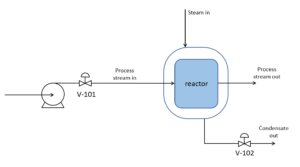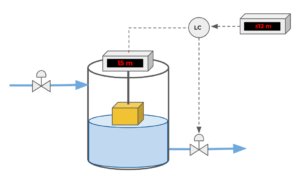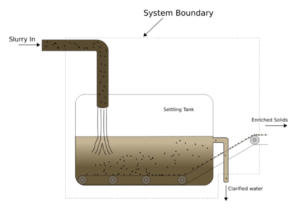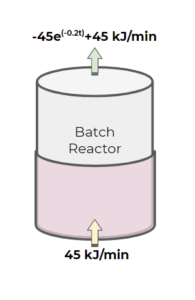# 44 Practice Exercises

## Multiple Choice Questions

Exercise: Separable Differential Equations

Which of the following is not a separable differential equation?

a) $\frac{t^{2}}{C_{A}} = 4*C_{A}\frac{dC_{A}}{dt}$

b) $\frac{t^{2}}{T} = 3*T \frac{dT}{dt}$

c) $\frac{dM_{A}}{dt} + e^{M_{A} + t} = 0$

d) $\frac{-2t + T^{2}}{2*T*t} = \frac{dT}{dt}$

### Solution

d) $\frac{-2t + T^{2}}{2*T*t} = \frac{dT}{dt}$

If you try to seperate this equation, you get: $2*t + T^{2} + 2*T*t*\frac{dT}{dt} = 0$. There is no way to isolate the T and t variables further.

How to separate the other choices:

a) \begin{align*}
\frac{t^{2}}{C_{A}}& = 4*C_{A}\frac{dC_{A}}{dt}\\
t^{2}dt&= 4*C_{A}^2\,dC_{A}
\end{align*}

b) \begin{align*}
\frac{t^{2}}{T} &= 3*T \frac{dT}{dt}\\
t^{2}dt &= 3*T^2dT
\end{align*}

c)\begin{align*}
\frac{dM_{A}}{dt} + e^{M_{A} + t} &= 0\\
\frac{dM_{A}}{dt} + e^{M_{A}}· e^{t} &= 0\\
e^{M_{A}}· e^{t} &= -\frac{dM_{A}}{dt} \\
e^{t}dt &=-\frac{1}{e^{M_{A}}}dM_{A}
\end{align*}

Exercise: Process Control Variables

Consider a mixing tank with 2 inlet streams and 1 outlet stream. Stream 1 is feeding the tank with a solution with a concentration of species A. We can change the flowrate of stream 1 using a valve. Stream 2 is feeding the tank with a solution with a concentration of species B, but we do not have control over stream 2’s flowrate or concentration (species B concentration fluctuates and is decided by an upstream process). We measure the concentration of the outlet stream and adjust our system accordingly. Which of the following variables is the disturbance variable?

a) Stream 1 flowrate

b) Stream 2 flowrate

c) Outlet stream flowrate

d) Outlet stream concentration

### Solution

b) Stream 2 flowrate

The flowrate of stream 2 is the best answer here because it is an input variable that is affecting our controlled variable (outlet stream concentration), but we do not have control over it. We must adjust our manipulated variable (stream 1 flowrate) to achieve our desired outlet flowrate or concentration.

Which of the following is not a steady-state system?

a) CSTR (continuous stirred-tank reactor) with constant flow in and out of all species

b) A filtration system that has fluid entering and exiting the system at constant volumetric flowrates

c) A bathtub filling up with water at the same rate as the drain empties the water

d) Heat exchanger in a process that maintains the process fluid at 50°C

### Solution

b) A filtration system that has fluid entering and exiting the system at the same rate

This system will accumulate a build-up of the filtered product. Although the flowrates entering and exiting the system are constant, the concentration will change over time due to the filter present.

Exercise: Methods to Decrease Reactor TemperatureWe have the following reactor with an endothermic reaction taking place inside the reactor. There is a heating jacket outside of the reactor that acts in a similar manner to a heat exchanger with steam as the utility stream. If the reactor temperature is currently lower than the desired temperature that is required to achieve the optimum yield from the reaction, how should we manipulate both valves to increase the temperature in the reactor?

a) V-101 and V-102 both open

b) V-101 and V-102 both close

c) V-101 opens; V-102 closes

d) V-101 closes; V-102 opens

### Solution

d) V-101 closes; V-102 opens

Since the reaction is endothermic, we want to slow down the reaction rate inside the reactor to increase the temperature, which can be achieved by closing V-101 to slow down the inlet flow of the reactant.

The heating jacket releases more heat into the reactor when there is more steam and less condensate in the jacket, due to most of the heat released comes from the condensation process. This can be achieved by opening V-102 to let out some of the condensates in the heating jacket.

Exercise: General Balance for Semi-Batch Reactor

Consider a semi-batch reactor that is initially filled with species A prior to the startup of the reactor. Species B is added to the reactor slowly until a stoichiometrically balanced (species A and B) amount is reached. There is a continuous outlet stream in the reactor. Species B reacts with species A with a conversion of 75% at the end of the process. The reaction is reversible.

$A + B \leftrightarrow C$

What terms in the general balance (IN – OUT + GEN – CON = ACC) are considered for species A in this system?

### Solution

• IN = 0: there is no A entering the reactor (it is already in the reactor prior to startup)
• OUT: some of reactant A does not react (75% conversion) or is produced from the reverse reaction, and therefore exits the system
• GEN: species A generated will come from the reversible reaction, where species C will form species A and B
• CON: species A will react and will be consumed along with species B to form species C

GEN – OUT – CON = ACC

Exercise: Level Control Loop

Consider the following control loop. The number indicated by the reader is the level of water (height of the water inside the tank), not the height from the top of the tank to the surface of the water. The exit valve is initially closed.What action will the level control take on the valve and why?

### Solution

The level controller will signal the valve to open to drain some of the water in the tank. The setpoint height in the tank is less than or equal to 12 m. Given that the tank water level is at 15 m, we must drain some of the water in the tank to bring the water level back to an acceptable range.

Exercise: Temperature Boundary Conditions

The change in temperature with respect to time for a system can be described by the following function, where the temperature(T) is in K and time(t) is in mins:

$\frac{dT}{dt} = 20e^{-0.2t}$

The temperature of the system is initially at 270 K. Solve for the time when the temperature change is equal to 95% of the temperature change t = 0 minutes to t → $\infty$

### Solution

\begin{align*}
T(t) &= \int 20e^{-0.2t}dt\\
T(t) &= -100e^{-0.2t} + C
\end{align*}

Our boundary condition is T(t=0) = 270 K. We can use this to solve for the constant in th temperature function.

\begin{align*}
270 &= -100e^{-0.2*0} + C\\
270 &= -100 + C\\
C &= 370 K
\end{align*}

Find the system temperature when time goes to infinity:

\begin{align*}
T(t=\infty) &= -100*0 + 370 K\\
T(t=\infty) &= 370 K
\end{align*}

Our temperature $T_{2}$ is going to be 95% of temperature change $T_{\infty} - T_{t=0}$ plus $T_{t=0}$:

\begin{align*}
T_{2} &= 0.95(370 K – 270 K) + 270 K\\
T_{2} &= 365 K
\end{align*}

Now, to find the time, we plug in our temperature $T_{2}$:

\begin{align*}
365 &= -100e^{-0.2t_{2}} + 370\\
-5 &= -100e^{-0.2t_{2}}\\
0.05 &= e^{-0.2t_{2}}\\
t_{2} &= \frac{ln(0.05)}{-0.2} = 15 mins
\end{align*}

Exercise: Mass Balance in a Filtration SystemSuppose we have the settling tank system above to separate solid particles from a waste stream. Given the following information:

• The slurry flows into the tank at a rate of 30 kg/hr with 10% solids
• The enriched solid stream carries solid out of the system at a rate of 2.5 kg/hr and contains 100% solids
• The clarified water stream flows out of the system at a rate of 27.5 kg/hr and contains 1% solids

We know that we need to stop the process and clean the tank when there is 500 kg of solids accumulated in the tank. If the tank is initially empty, what is the maximum time before we must clean the tank up?

### Solution

We write each term in the mass balance in terms of the slurry and simplify the mass balance:

• $IN = 30\frac{kg}{hr}×10\text{%}=3\frac{kg}{hr}$
• $OUT = 2.5\frac{kg}{hr}×100\text{%}+27.5\frac{kg}{hr}×1\text{%}=2.775\frac{kg}{hr}$
• $GEN = 0$
• $CON =0$
• $ACC = IN-OUT$

Since the tank is initially empty, we know that the $M_{i}=0kg$ when t=0 hr, so we can solve the mass balance to find t when $M_{i}=500kg$.

\begin{align*}
\int_{t_i=0hr}^{t_f}3\frac{kg}{hr}dt-\int_{t_i=0hr}^{t_f}2.775\frac{kg}{hr}dt&=\int_{M_i=0kg}^{M_f=500kg}dM\\
3\frac{kg}{hr}×(t_f-0)hr-2.775\frac{kg}{hr}×(t_f-0)hr&=(500-0)kg\\
t_f×0.225\frac{kg}{hr}&=500kg\\
t_f &=2222hr (\cong 93days)
\end{align*}

Exercise: Mass Balance in a Filtration SystemFor the same settling tank system, suppose we have the following information instead:

• The slurry flows into the tank at a rate of 30kg/hr with 10% solids
• The enriched solid stream carries solid out of the system at a rate of 2.5 kg/hr and contains 100% solids
• The clarified water stream carries out 0.1% of the total mass of solids in the tank every hour

Express each term in the mass balance for the mass of the solids inside the tank. Construct the balance equation to solve for the time when 500kg of solids accumulated in the tank. You do not need to solve the equation.

### Solution

Let M be the total mass of solids in the tank. We write each term in the mass balance in terms of the slurry and simplify the mass balance:

• $IN = 30\frac{kg}{hr}×10\text{%}=3\frac{kg}{hr}$
• $OUT = 2.5\frac{kg}{hr}×100\text{%}+0.1M\frac{kg}{hr}=(2.5+0.1M)kg/hr$
• $GEN = 0$
• $CON =0$
• $ACC = IN-OUT$

Since the tank is initially empty, we know that the $M_{i}=0kg$ when t=0hr, so we can solve the mass balance to find t when $M_{i}=500kg$.

\begin{align*}
\int_{t_i=0hr}^{t_f}3\frac{kg}{hr}dt-\int_{t_i=0hr}^{t_f}(2.5+0.1M)\frac{kg}{hr}dt&=\int_{M_i=0kg}^{M_f=500kg}dM
\end{align*}

Because M is also a function of t, this equation is not separable, see we don’t need to solve it in this course.

Exercise: Batch Reactor with Unsteady-state Energy Balance

A batch reactor is used for a reaction that requires a high temperature prior to adding a catalyst to the system. A heated jacket is used to heat the batch reactor. The heat jacket provides heat to the batch reactor at a rate of $45\frac{kJ}{min}$. The batch reactor vessel is losing energy at a rate described by the following function, where energy is in $\frac{kJ}{min}$ and time is in mins:

$-45e^{-0.2t}+45$

The accumulation of heat in the system (batch reactor) can be described by $C_{P}*m \frac{dT}{dt}$, where $C_{P} = 0.38 \frac{kJ}{kg*K}$ and the mass of the reactor is $10 kg$. The reactor is initially at 300 K.a) Write out the overall energy balance, with each of the terms in the IN-OUT+GEN-CON=ACC formula.

b) At what time will the reactor reach a temperature of 350 K?

### Solution

a) For each of the energy balance terms, we have:

• $IN = 45\frac{kJ}{min}$
• $OUT = (-45e^{-0.2t}+45)\frac{kJ}{min}$
• $GEN = 0$
• $CON =0$
• $ACC = C_{P}*m \frac{dT}{dt} = 0.38 \frac{kJ}{kg*K}* 10 kg * \frac{dT}{dt}$

b) Once again, we solve the energy balance:

\begin{align*}
45\frac{kJ}{min} – (-45e^{-0.2t}+45)\frac{kJ}{min} &= C_{P}*m \frac{dT}{dt}\\
45\frac{kJ}{min} + (45e^{-0.2t} – 45)\frac{kJ}{min} &= 0.38 \frac{kJ}{kg*K}* 10 kg * \frac{dT}{dt}\\
45e^{-0.2t}\frac{kJ}{min}&= 0.38\frac{kJ}{kg*K}*10 kg*\frac{dT}{dt}\\
45e^{-0.2t}\frac{kJ}{min}&= 3.8\frac{kJ}{K}*\frac{dT}{dt}
\end{align*}

We know that the temperature of the batch reactor is initially at 300 K. Therefore, our first boundary condition is (t1=0 mins, 300 K). Our second boundary condition will be (t2, 350 K). We can now separate our differential equation and take the integral of both sides:

\begin{align*}
\int_{0 mins}^{t_{2} mins} 45e^{-0.2t}\frac{kJ}{min}*dt &= \int_{300 K}^{350 K} 3.8 \frac{kJ}{K}* dT\\
(\frac{-45}{0.2}e^{-0.2t_{2}} + \frac{45}{0.2}e^{-0.2*0})\frac{kJ}{min} &= 3.8\frac{kJ}{K}*(350 K – 300 K)\\
\frac{45}{0.2}(1-e^{-0.2*t_{2}}) \frac{kJ}{min}&= 190 kJ\\
225(1-e^{-0.2*t_{2}}) \frac{kJ}{min}&= 190 kJ\\
(1-e^{-0.2*t_{2}}) &= \frac{190 kJ}{225 \frac{kJ}{min}}\\
(1-e^{-0.2*t_{2}})&= 0.84\\
e^{-0.2*t_{2}}&= 0.16\\
-0.2*t_{2}&= ln(0.16)\\
t_{2} &= \frac{ln(0.16)}{-0.2}\\
t_{2}&= 9.2 minutes
\end{align*}